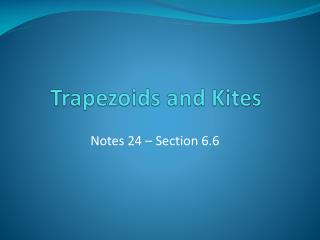DownloadDownload PresentationTrapezoids and Kites

# Trapezoids and Kites

Download Presentation## Trapezoids and Kites

- - - - - - - - - - - - - - - - - - - - - - - - - - - E N D - - - - - - - - - - - - - - - - - - - - - - - - - - -
##### Presentation Transcript

1. Trapezoids and Kites Notes 24 – Section 6.6

2. Essential Learnings • Students will understand and be able to recognize and apply the properties of trapezoids and kites.

3. Trapezoid • A trapezoid is a quadrilateral with exactly one pair of parallel sides. Base Leg Leg Base

4. Isosceles Trapezoid • If the legs of a trapezoid are congruent then it is an isosceles trapezoid. Base Leg Leg Base

5. Isosceles Trapezoids • If a trapezoid is isosceles, then each pair of base angles is congruent. A B D C

6. Isosceles Trapezoids • If a trapezoid has one pair of congruent base angles, then it is an isosceles trapezoid. A B D C

7. Isosceles Trapezoids • A trapezoid is isosceles if and only if its diagonals are congruent. A B D C

8. Example 1 JKLM is an isosceles trapezoid. Find m∠K and x. J K 2x 14 112º L M

9. Midsegment of a Trapezoid • The midsegment of a trapezoid is a segment that connects the midpoints of the legs of the trapezoid.

10. Trapezoid Midsegment Theorem • The midsegment of a trapezoid is parallel to each base and its measure is one half the sum of the lengths of the bases. x m y

11. Example 2 For trapezoid JKLM, P and Q are midpoints of the legs. If JK = 18 and PQ = 28, find LM. J K P Q M L

12. Kite • A kite is a quadrilateral with exactly two pairs of consecutive congruent sides.

13. Kites • If a quadrilateral is a kite, then its diagonals are perpendicular. • One diagonal is bisected. B A C D

14. Kites • If a quadrilateral is a kite, then exactly one pair of opposite angles is congruent.

15. Example 3 If WXYZ is a kite, find m∠XYZ. W Z 48º 110º X Y

16. Example 4 If MNPQ is a kite, find NP. P 4 N Q 7 11 M

17. Example 5 If m∠WXY = 72, m∠WZY = 4x and m∠ZWX = 10x, find m∠ZYX. W U Z X Y

18. Assignment Pages 440-442: 8-11, 17, 19, 21, 26, 27, 35-39, 41, 43, 49, 50, 53-57 Unit Study Guide 4 – Due Monday (12/10)